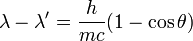# Compton scattering

Compton scattering is the scattering of photons by (free) electrons. It is a phenomenon in physics, first observed and explained by Arthur Holly Compton. His explanation is based on the assumption that X-rays consist of particles with well-defined relativistic kinetic energy and momentum. If a stream of X-rays is fired at electrons the rays are scattered and the scattered radiation will have smaller frequency (and longer wavelength) than the incident radiation. The change in wavelength is dependent on the angle through which the radiation is scattered. Arthur Compton earned the 1927 Nobel Prize for Physics for his discovery.

##  Compton Scattering Formula

If the radiation is scattered at an angle θ, and λ and λ'are the incident and scattered wavelengths then:$\lambda - \lambda' = \frac{h}{mc} (1-\cos \theta)$

The angle θ is the angle between incident and scattered rays.